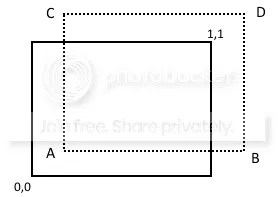So you are creating this 2D or 3D scene. and you have an image you’d like to use to clothe a building, or the ground (eg grass, dirt etc). Because the image is smaller than the area you want to cover with it, you need to tile the image (ie lay multiple copies of the image next to each other).

There are at least two problems here.

### Tiling images

One is how to tile images, and I dealt with that back here, using a simple shader.

### Creating a seamless image

The other problem is how to create an image that can be tiled seamlessly. By that, I mean that when you look at the result, you can’t tell where the tile start and end. This means the left and right sides need to fit together without an obvious join, and the same applies for the top and bottom sides.

There are at least two ways to do this outside of Codea.

• search the internet for “seamless” textures of whatever image you want
• Photoshop and Blender (free) can make images seamless, I understand

However, I have been frustrated in the past in not being able to find just the right image, in seamless form. So I wondered if Codea could do it.

This next section is very similar to the last part of my previous post on noise. We use a shader to create a seamless image from the original image, by making a copy of the image and drawing on it with the shader.

Suppose the image we are tiling, is the solid rectangle below, and our shader is drawing at the point A shown.We interpolate between the noise value at A and the points B, C and D shown above. This means that as you approach an edge, it gets closer to the value at the opposite edge, creating a seamless fit.

```
//B, C and D are outside the image, so we'll wrap around and start at the beginning again
vec4 A = texture2D(vTexCoord);
vec4 B = texture2D(vec2(1.0-vTexCoord.x,vTexCoord.y));
vec4 C = texture2D(vec2(vTexCoord.x,1.0-vTexCoord.y));
vec4 D = texture2D(1.0-vTexCoord);
//calculate interpolation factor on x and y axis
float xx = 1.0 - pow(vTexCoord.x,p);  //see note on pow below
float yy = 1.0 - pow(vTexCoord.y,p);
vec4 AB = mix(A,B,xx); //interpolate A,B on x axis
vec4 CD = mix(C,D,xx); //interpolate C,D on x axis
vec4 u = mix(AB,CD,yy); //interpolate results on y axis
u.w=1.0;  //set alpha to full value
gl_FragColor = u;
```

Where do we get colour values for B, C and D, which fall outside our image? I’ve assumed our image is mirrored to the right and above.

We then interpolate the four points together. Note the power function pow used to calculate the interpolation factors xx and yy. If you simply want linear interpolation, you set the parameter p to 1. But if you want a non linear interpolation which only happens very close to the edge, you can set p higher.

A high value of p helps get rid of obvious edges. However, if there is a big brightness contrast between opposite edges, a lower p value works better.

I should note that I didn’t invent this interpolation method, but since it was created using noise (which has values at B, C and D and doesn’t have to wrap around), I did have to decide what to do for B, C and D, and I also introduced the power function to improve the results.

The video below shows for several different images, the effect of

1. first tiling the raw image, showing the obvious joins
2. showing the effect of different levels of p, showing how the best value changes for different images

While it is still obviously better to find professionally made seamless images, this approach works pretty well.

EDIT: instead of using a power function, the code below interpolates only very close to the join, and produces pretty good results for all the images I tested. Replace the definitions of xx and yy in the shader with these lines.

```float z=0.90;
xx=1.0-clamp((vTexCoord.x-z)/(1.0-z),0.0,1.0);
yy=1.0-clamp((vTexCoord.y-z)/(1.0-z),0.0,1.0);
```